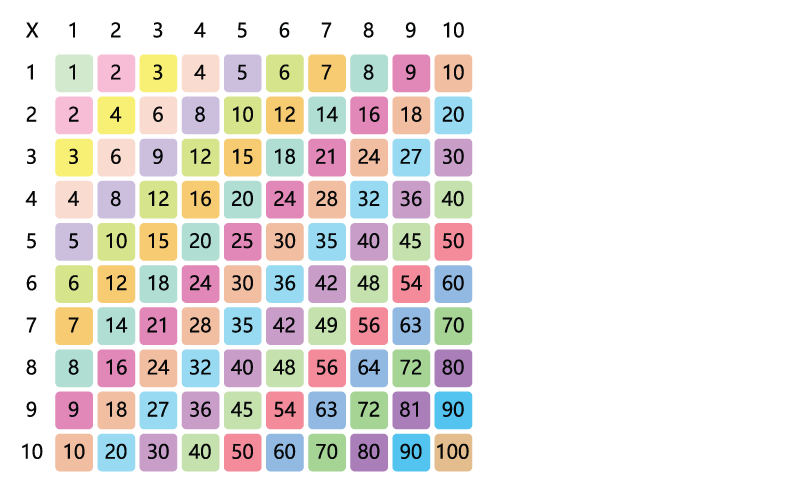Square numbers look like this , , , and so on...

When you square a number you multiply it by itself.

Take a look at the multiplication table below.Example:

Two squared 2² = 2 x 2 = 4

Three squared 3² = 3 x 3 = 9

Four squared 4² = 4 x 4 = 16

Five squared 5² = 5 x 5 = 25